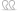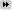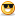Originally Posted by Liuprecious soul = 50 frags
conversion rate 5 derby frags = 1 prec soul frag

50 * 5 = 250

=> 1.25 derby demon souls = 1 prec soul

sac val of sac hearts at max lvl:
1* = 1000
3* = 1500
5* = 4000

assuming each precious soul drops with 1/6 (which is overestimating the sac val)
expected sac val = 1/6 * (1k + 1.5k + 4k) = 1/6 + 6.5k = 1083

sac val of derby demons at max lvl:
4* = 2400

1.25 * 2.4k = 3k

3k > 1083

and 1083 is already an overestimation due to the assumption of uniformly distributed pulls.
so yeah, unless i did a stupid mistake then only convert your leftovers after the event has terminated.

of course you have to take monetary gains into account as well. so lets add them up (assuming again a uniform dist again)

sell val of idols:
1* = 50k
3* = 300k
5* = 1m (not sure if its actually 1m, i didn't get them often enough to memorize it ><)

1/6 * (1.35m) = 225k

which is overestimating the monetary gain.

then you have 3k sac val vs 1083 sac val + 225k gold

if you want the proper expected values just replace the uniform probabilities with the actual probabilities which should be decreasing the expected values.Wow, really mad math there! Would like to hear the optimum calculations when it is sacrifice event